© PINOYGSM Would you like to react to this message? Create an account in a few clicks or log in to continue.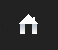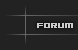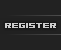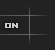This is the FIRST PINOY GSM forum in the Philippines. The Number "One PinoyGSM dot com" PINOY, Global System for Mobile communication (GSM) is a globally accepted standard for digital cellular communication. and security tools for the computer systems and networks. ^^^ PinoyGSM is the name of a standardization group. ^^^ Be One Of Us! PINOYGSM Forum. ^^^ We Are Hackers Who Would Go Beyond Technology ^^^
 Time in Philippines
 Who’s HACKER?We’re Hiring toktok Driver# Program to determine area,perimeter of geometric shapes using coordinates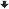AuthorMessage
kulit™Join Date : 2008-04-25
Location : Malabon City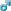Age : 37
Cell Phone # : 09273560700
Posts : 367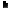Subject: Program to determine area,perimeter of geometric shapes using coordinates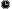August 20th 2012, 14:53 #include #include void main()//Program to determine area and perimeter of geometric shapes using the x,y coordinates {int choice,x1,y1,x2,y2,x3,y3,x4,y4; float a,b,c,r,A,P,l,w;// user chooses shape.printf("Welcome to Cartesian geometry! Please choose your shape by entering its number:\n");printf("rectangle -> enter 1\n");printf("circle -> enter 2\n");printf("triangle -> enter 3\n");printf("to exit -> enter 4\n");scanf("%d", &choice); if (choice==1) { prinf("Please enter the coordinates for the rectangle from bottom left to right, then top right to left"); printf("\nenter value for x1:"); scanf("%d", &x1); printf("enter value for y1:"); scanf("%d", &y1); printf("enter value for x2:"); scanf("%d", &x2); printf("enter value for y2"); scanf("%d", &y2); printf("enter value for x3:"); scanf("%d", &x3); printf("enter value for y3:"); scanf("%d", &y3); printf("enter value for x4:"); scanf("%d", & x4); printf("enter value for y4:"); scanf("%d", &y4); l = (((x1-x2)^2 + (y1-y2)^2))^(1/2); w = (((x1-x4)^2 + (y1-y4)^2))^(1/2); A=l*w; printf("the area is %f\n", A); P=2*(l+w); printf("the perimeter is %f", P); }if (choice==2) { printf("Please enter the centre of the circle, and a point on the circle\n"); printf("enter value for x1\n"); scanf("%f", &x1); printf("enter value for y1\n"); scanf("%f", &y1); printf("enter value for x2\n"); scanf("%f", &x2); printf("enter value for y2\n"); scanf("%f", &y2); r=(((x2-x1)^2 + (y2-y1)^2))^(1/2); A=3.14*(r)^2; printf("\nThe Area of the triangle is %f", A); P=2*3.14*r; printf("The Perimeter of the triangle is %f", P); if (choice==3) { printf("Please enter the x,y coordinates of the triangle starting with the bottom left to right, then the top:"); scanf("%f", &x1); printf("\nenter value for y1"); scanf("%f", &y1); printf("please enter value for x2"); scanf("%f", &x2); printf("please enter value for y2"); scanf("%f", &y2); printf("enter value for x3"); scanf("%f", &x3); printf("enter value for y3"); scanf("%f", &y3); a=((x2-x1)^2 + (y2-y1)^2))^(1/2); b=((x3-x2)^2 + (y3-y2)^2))^(1/2); c=((x3-x1)^2 + (y3-y1)^2))^(1/2); A=(.25)*(((a^2+b^2+c^2))^(2) - (a^4+b^4+c^4))^(1/2); printf("The area is %f", A); P=a+b+c; printf("The perimeter is %f", P); if (choice>=4) { return 0; }}_________________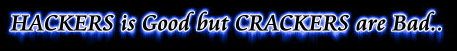www.1pinoygsm.com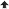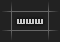Program to determine area,perimeter of geometric shapes using coordinatesPage 1 of 1
 Similar topics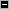» C Program Language (Area Of Circle, Triangle and Square)
» BULACAN AREA
» Compute The Area Of A Circle In C++
» 5130 program
» program in c to calculate age

Permissions in this forum:You cannot reply to topics in this forumJump to: Select a forum||--CHAPTER TRANSFORMING    |--Metro Manila - NCR    |   |--Malabon Chapter    |   |--Mandaluyong Chapter    |   |--Marikina Chapter    |   |--Muntinlupa Chapter    |   |--Navotas Chapter    |   |--Paranaque Chapter    |   |--Pasay Chapter    |   |--Quezon City Chapter    |   |--San Juan Chapter    |   |--Taguig Chapter    |   |--Valenzuela Chapter    |       |--Region I    |   |--Ilocos Norte Chapter    |   |--Ilocos Sur Chapter    |   |--La Union Chapter    |   |--Pangasinan Chapter    |       |--Region II    |   |--Batanes Chapter    |   |--Cagayan Chapter    |   |--Isabela Chapter    |   |--Quirino Chapter    |       |--Region III    |   |--Aurora Chapter    |   |--Bataan Chapter    |   |--Bulacan Chapter    |   |--Nueva Ecija Chapter    |   |--Pampanga Chapter    |   |--Tarlac Chapter    |   |--Zambales Chapter    |       |--Region IV -A    |   |--Cavite Chapter    |   |--Laguna Chapter    |   |--Batangas Chapter    |   |--Rizal Chapter    |   |--Quezon Chapter    |       |--Region IV -B    |   |--Marinduque Chapter    |   |--Mindoro Occidental Chapter    |   |--Mindoro Oriental Chapter    |   |--Palawan Chapter    |   |--Romblon Chapter    |       |--Region V    |   |--Albay Chapter    |   |--Camarines Norte Chapter    |   |--Camarines Sur Chapter    |   |--Catanduanes Chapter    |   |--Masbate Chapter    |   |--Sorsogon Chapter    |       |--CAR (Cordillera Administrative Region)    |   |--Benguet Chapter    |   |--Abra Chapter    |   |--Bontoc Chapter    |   |--Ifugao Chapter    |   |--Apayao Chapter    |   |--Mountain Province Chapter    |       |--Region VI    |   |--Aklan Chapter    |   |--Boracay island Chapter    |   |--Antique Chapter    |   |--Capiz Chapter    |   |--Guimaras Chapter    |   |--Iloilo Chapter    |   |--Negros Occidental Chapter    |       |--Region VII    |   |--Bohol Chapter    |   |--Cebu Chapter    |   |--Negros Oriental Chapter    |   |--Siquijor Chapter    |       |--Region VIII    |   |--Catarman Chapter    |   |--Calbayog City Chapter    |   |--Catbalogan City Chapter    |   |--Borongan City Chapter    |   |--Dolores Chapter    |   |--Tacloban City Chapter    |   |--Ormoc City Chapter    |       |--Region IX    |   |--Zamboanga Del Norte Chapter    |   |--Zamboanga Del Sur Chapter    |   |--Zamboanga Sibugay Chapter    |       |--Region X    |   |--Bukidnon Chapter    |   |--Camiguin Chapter    |   |--Lanao Del Norte Chapter    |   |--Misamis Occidental Chapter    |   |--Misamis Oriental Chapter    |       |--Region XI    |   |--Compostela Valley Chapter    |   |--Davao del Norte Chapter    |   |--Davao City Chapter    |   |--Davao del Sur Chapter    |   |--Davao Oriental Chapter    |       |--Region XII        |--North Cotobato Chapter        |--South Cotobato Chapter        |--Sarangani Chapter        |--Sultan Kudarat Chapter